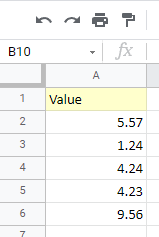# How to Round Numbers Without Formula in Excel & Google Sheets

This tutorial demonstrates how to round numbers without a formula in Excel and Google Sheets.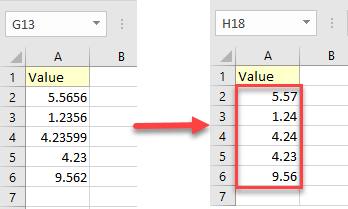## Round Numbers

Say you have the following data set with values in Column A.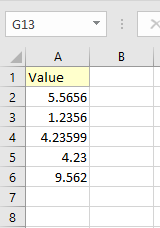You could use the ROUND Function to round the values in your dataset, but Excel also has a few ways to round up, round down, or chop off numbers without a formula.

### Change the Number of Decimals

One rounding method is to use the decrease or increase decimal buttons.

#### Round With Decrease Decimal

1. First, select the cells with values you want to round (A2:A6).
2. Then in the Ribbon, go to Home.
3. In the Number group, click on the Decrease Decimal icon. The decimal places go down by the number of times you click on this button. In this example, clicking two times rounds all values to two decimal places.As a result, the numbers of decimal places decreases.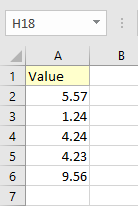#### Increase Decimal Places

To increase decimal places, you can change how a value is rounded by increasing the number of decimal places. Say you want to increase decimal places from 2 to 5.

1. First, select the cells (A2:A6).
2. Then in the Ribbon, go to Home.
3. Press Increase Decimal (here, three times).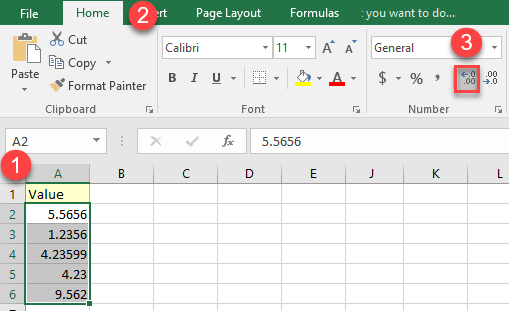As a result, the number of decimal places increases.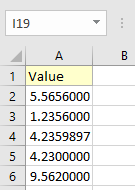## Round With Number Formatting

1. To round numbers using a number format, (1) select the cells with values you want to round (A2:A6). Then in the Ribbon, (2) go to Home, and (3) select the Number Format icon in the lower right corner.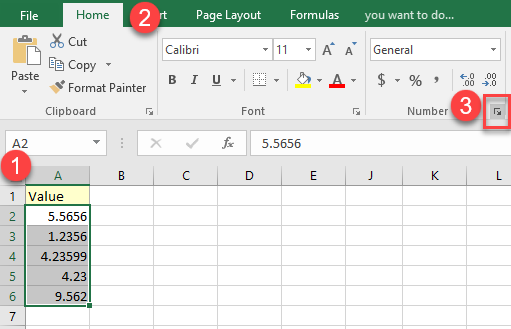1. This opens the Format Cells window. In it, (1) select the Number tab, then under Category, (2) choose Number. (3) In the Decimal places box, specify the number of decimal places. (4) Press OK.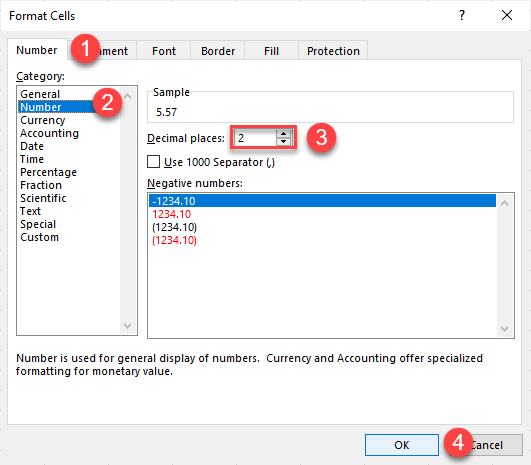This rounds the values to the number of decimals you selected.### Custom Format

You can also round numbers with a custom format with these steps:

1. Select the cells with values you want to round (A2:A6), and right-click them. From the list, choose Format Cells…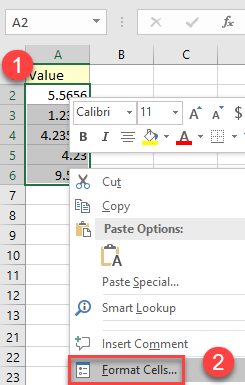1. In the Format Cells window, click on (1) Number and (2) choose Custom from the list. In the Type box (3) enter the format you want.
In this example, entering 0.00 formats the values to two decimal places.
(4) Press OK.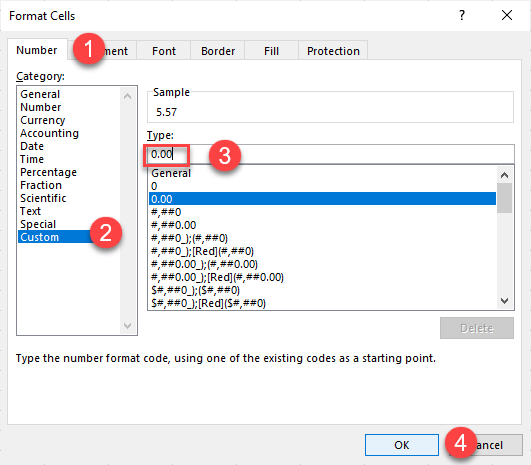As a result, all selected values have two decimal places.## Round Numbers in Google Sheets

In Google Sheets, you can round numbers using a custom number format with these steps:

1. Select the cells with values you want to round. Then in the Toolbar, click the More Formats icon.
From the drop-down list, choose More Formats > Custom number format.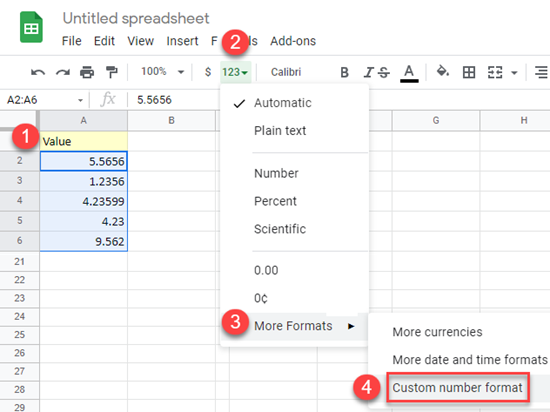1. In the Custom number formats window, enter the format you want.
In this example, entering 0.00 formats the values to two decimal places.
Click Apply.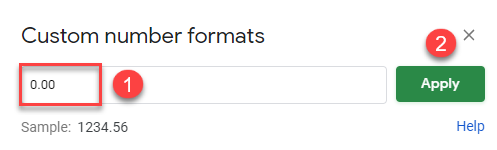As a result, all selected values are displayed as numbers with two decimal places.